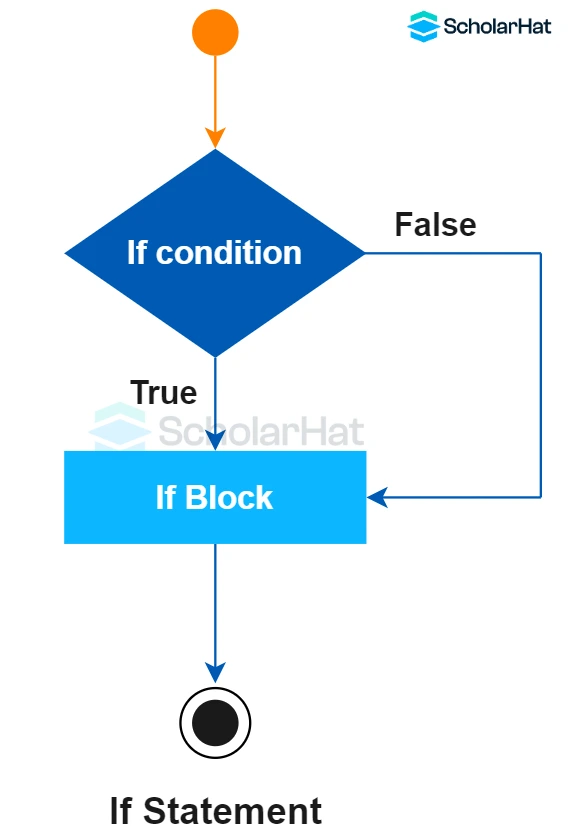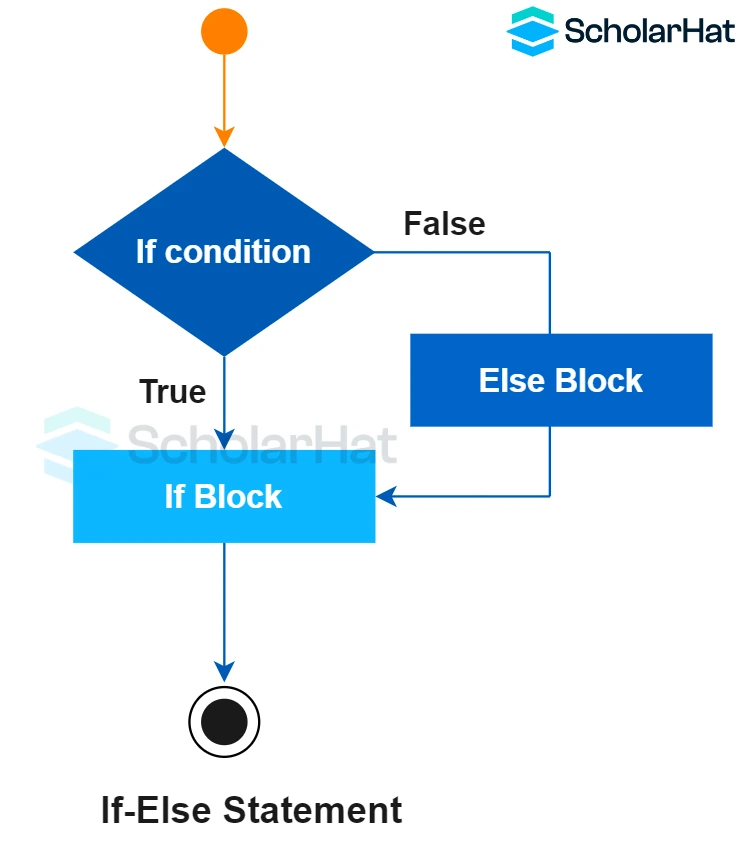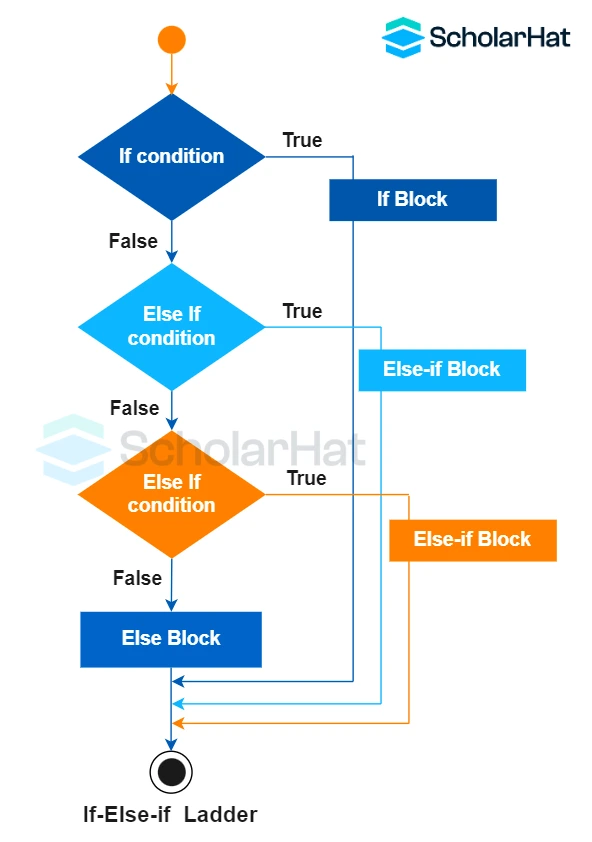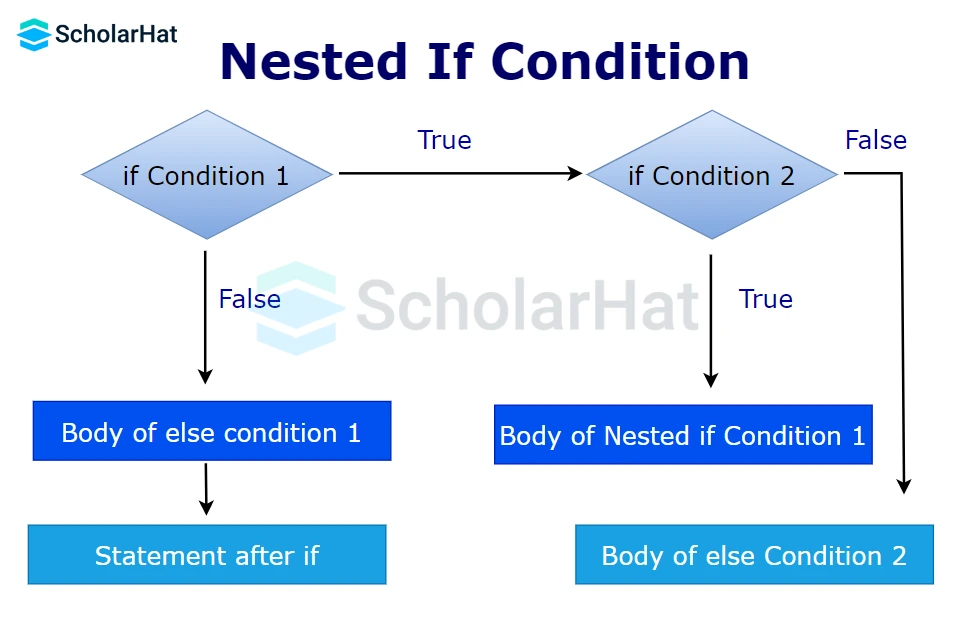# Conditional Statements in C: if, if..else, Nested if

23 Nov 2023
Beginner
453 ViewsLearn via Video Course & by Doing Hands-on Labs

## Conditional Statements in C: An Overview

If you are a programmer, then you already know how to use `if...else` statements. If not, it's time to learn! Understanding `if...else` statements is essential, especially for beginners. After reading this article in the C tutorial, you will have a fundamental understanding regarding use of `if...else` statement in C programming. However, if you're looking to solidify your knowledge and demonstrate your proficiency in C programming, consider enrolling in ourC certification course.

## Conditional Statements in C

The conditional statements are also known as decision-making statements. They are of the type `if statement`, `if-else`, `if else-if ladder`, `switch`, etc. These statements determine the flow of the program execution.

## Why Conditional Statements?

• Dynamically controls the manner of program execution.
• Useful in error and exception handling.
• Check the flow of your program.
• Helps to manage the state of your program.
• Helpful in performing complex calculations.

## Types of Conditional Statements in C

In this article we'll discuss the first kind of conditional statement i.e. `if...else` statements in C. We'll discuss the `switch` statement in the next tutorial switch Statement in C.

## if … else statements

`if-else` is the first kind of control statement in C. In this, the operations are performed according to some specified condition. The statements inside the body of the `if` block get executed if and only if the given condition is true.

## Variants of if-else statements in C

We have four variants of `if-else` statements in C. They are
1. `if` statement in C
2. `if-else` statement in C
3. `if else-if ladder` in C
4. `nested if` statement in C

### if statement in C

When you want to print any code only upon the satisfaction of a given condition, go with the simple `if` statement.Syntax

``````if(test condition){
//code to be executed
}
``````

#### Example

``` ``` #include<stdio.h>
int main(){
int num=8;
if(num%2==0){
printf("%d is even number",num);
}
return 0;
}
``````
• The above code prints the even number.
• The `if` statement checks if the user input is odd or even.
• If the number is even, the statement inside the `if` block gets printed.
• If the given input is odd, nothing gets printed.

#### Output

``````8 is even number
``````

### if-else statement in C

It is an extension of the `if` statement. Here, there is an `if` block and an `else` block. When one wants to print output for both cases - `true` and `false`, use the `if-else` statement.#### Syntax

``````if(test condition){
//code to be executed if condition is true
}else{
//code to be executed if condition is false
}
``````

#### Example

``` ``` #include <stdio.h>
int main() {
int num = 10;
if(num < 5) {
printf("Number is less than 5\n");
}
else if(num < 10) {
printf("Number is between 5 and 9\n");
}
else {
printf("Number is 10 or greater\n");
}
return 0;
}
``````
• In the above code, the `if` condition checks if the `num` is less than 5
• The given number 10 is greater than 5.
• Therefore, the `if` block gets skipped.
• The statement inside the `else` block gets printed.

#### Output

``````Number is 10 or greater
``````

### if else-if ladder in C

It is an extension of the `if-else` statement. If we want to check multiple conditions if the first “`if`” condition becomes false, use the `if else-if ladder`. If all the `if` conditions become false the `else` block gets executed.#### Syntax

``````if(test condition1){
//code to be executed if condition1 is true
}else if(test condition2){
//code to be executed if condition2 is true
}
else if(test condition3){
//code to be executed if condition3 is true
}
...
else{
//code to be executed if all the conditions are false
}
``````

#### Example

``` ``` #include <stdio.h>
int main(){
int number=5;
if(number==20){
printf("number is equals to 20");
}
else if(number==50){
printf("number is equal to 50");
}
else if(number==100){
printf("number is equal to 100");
}
else{
printf("number is not equal to 20, 50 or 100");
}
return 0;
}
``````
• In the given code, the program evaluates multiple conditions.
• If the first `if` condition becomes false, the control goes to the `else-if` statements.
• If all the conditions fail, the statement inside the `else` block gets printed.

#### Output

``````number is not equal to 20, 50 or 100
``````

### Nested if…else statement in C

We can include an `if-else` block in the body of another `if-else` block. This becomes a `nested if-else` statement.#### Syntax

``````if(test condition1)
{ //if condition1 becomes true
if(test condition1.1)
{ //code executes if both condition1 and condition 1.1 becomes true
}
else
{ //code executes if condition1 is true but condition 1.1 is false
}
}
else
{ //code executes if condition1 becomes false
}``````

#### Example

``` ``` #include <stdio.h>
int main()
{
int a = 10;
int b = 5;
if (a > b)
{
// control goes to the nested if
if (a % 2 == 0) {
printf("%d is even\n",a);
}
else {
printf("%d is odd\n",a);
}
}
else
{
printf("%d is not greater than %d\n",a,b);
}
return 0;
}
``````
• In the above code, the first `if` statement checks whether `a>b`.
• If `a` is greater than `b`, the `nested if` statement is checked.
• If the `nested if` condition is `false`, the `else` statement in the `nested if` block gets executed.
• If the first `if` statement evaluates to `false`, the `nested` `if` block gets skipped and the `else` block executes.

#### Output

``````10 is even
``````
##### Summary

`if...else` statements in C are a crucial part of writing an efficient program. They help to organize code and can account for a variety of conditions using comparison operators and logical operators. If used correctly, `if...else` statements can save your time from writing multiple lines of code. Knowing how to use them properly is thus essential for any programmer. You can also check out our C Certification Trainingtotest your knowledge and get comfortable with using `if...else` statements.

Share Article
Similar Articles